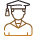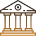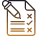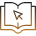# Why Should I Learn About Electric Power?

###### BySafeer PP###### Synopsis

Students often are piqued to learn things better when they can see or experience it in real life. This is especially true about concepts in science and maths. Electric power is an important concept for high school. The NCERT Class 10 science book has a chapter on electrical power. Here are some of the practical or real life uses of learning about electrical power.###### Synopsis

Students often are piqued to learn things better when they can see or experience it in real life. This is especially true about concepts in science and maths. Electric power is an important concept for high school. The NCERT Class 10 science book has a chapter on electrical power. Here are some of the practical or real life uses of learning about electrical power.

In Class 10 NCERT and most of the state board syllabus students learn about electricity. Electricity is the main source of energy. We use it at home, in schools and offices too. Talking about how electrical power helps us even in mundane day-to-day activities may spark an interest among students to learn about the subject in detail. Here the discussion is on a particular topic electric power and energy coming under the chapter of electricity.

## What Is Electric Power?

Electrical power is the rate at which the electrical energy is dissipated or consumed. While purchasing an electrical equipment or device you can see power ratings on it. For a ceiling fan you can see 120 watt, for a led bulb 9 watts or 12 watts, Microwave oven 1200 watt, air conditioner 1500 watt and more. What does this electrical power rating mean? What is the use of it? To understand the use of these power ratings students should know the concept of power and commercial unit of energy.

## Commercial Unit Of Electric Energy Consumption

Electrical power is expressed in Watts(W). For commercial calculations, kilowatts is useful. 1 kilowatt is equal to 1000 watts. The commercial calculation of energy is done in kiloWatt hour or kWh. Let us see what is kWh

1 kWh = 1000 W x 3600 seconds = 3600000 or 3.6 x 106 Joule=1 unit of energy commercially.

That is if an equipment with power rating of 1000 W or 1 kW is working for an hour it consumes 1 unit of electrical energy. This is where the importance of electrical power ratings comes. That is power rating is useful to calculate the energy consumption and thus helps in calculation of electric bill.

Also Read| How To Prepare For NTSE

## Calculation Of Electric Bill

Let us see how much a room contributes to electric bills. For example if your room has a ceiling fan of rating 120W, a bulb of rating 12 W and a mobile charger of 5 W. Let’s say you switch on the bulb and fan for 10 hours and charge your mobile for an hour. Then the contribution of your room to the electricity bill can be calculated as follows.

Calculation

Energy consumed by fan = (120/1000) kW x 10 h=0.12 x 10 kWh=1.2 units per day

Note: The division by 1000 is done to convert power to kW.

Energy consumption by bulb=(12/1000)kw x 10 h =0.12 kwh=0.12 units/day

Energy consumed by mobile charger=(5/1000)kW x 1h = 0.005 kWh=0.005 unit/day.

If the electricity bill is calculated for a month(30 days) then the total unit consumed by your room =30 (1.2+0.12+.005)=30(1.325)=39.75units. If the average price per unit is 5 rupees, then the electricity bill for your consumption = 39.75 x 5=198.75 rupees. This is the amount of consumed electricity. You may have a metre charge and a fixed amount in the bill apart from this. The electricity charges differ state wise. This is just an example.

By knowing the importance of electrical energy consumption, we can use this knowledge to calculate the total electric bill and can plan for a reduced bill by looking at the power rating of each equipment and how much time it is used and is it necessary to use it for that particular time duration.

The above given calculation is a simple one without including any other factors. The calculations are done for a high school student to understand the use of electric power in daily life. Try to save energy by using the equipment only when it is required. We must take care to switch off lights, AC and fan when going out of the room to save energy. Saving energy is a great start to sustainable living.

• Technology
• Energy
• Nature
• Quantity
• Electricity
• Electric power
• Electromagnetism
• Physical quantities
• Electrical engineering
• Metrology

## Careers360 helping shape your Career for a better tomorrow#### 250M+

Students#### 30,000+

Colleges#### 500+

ExamsE-Books